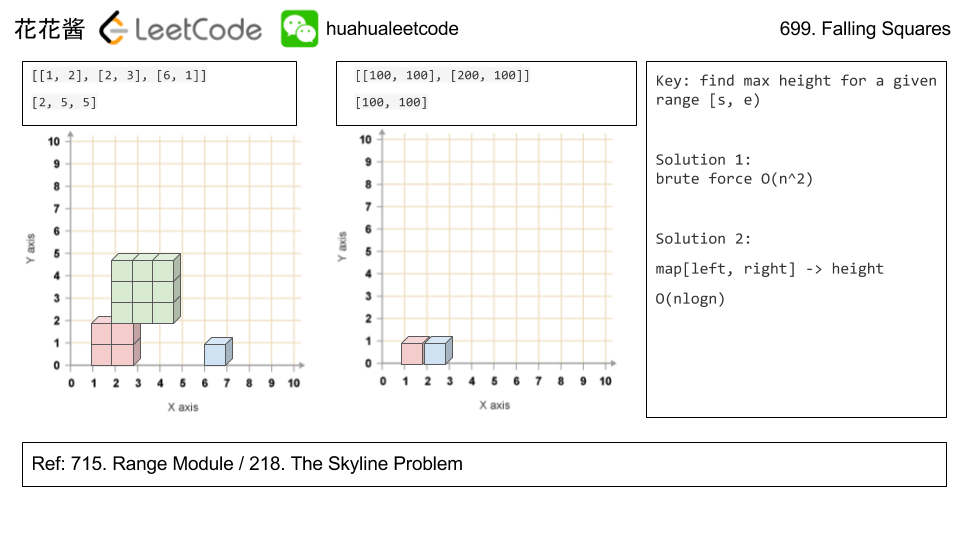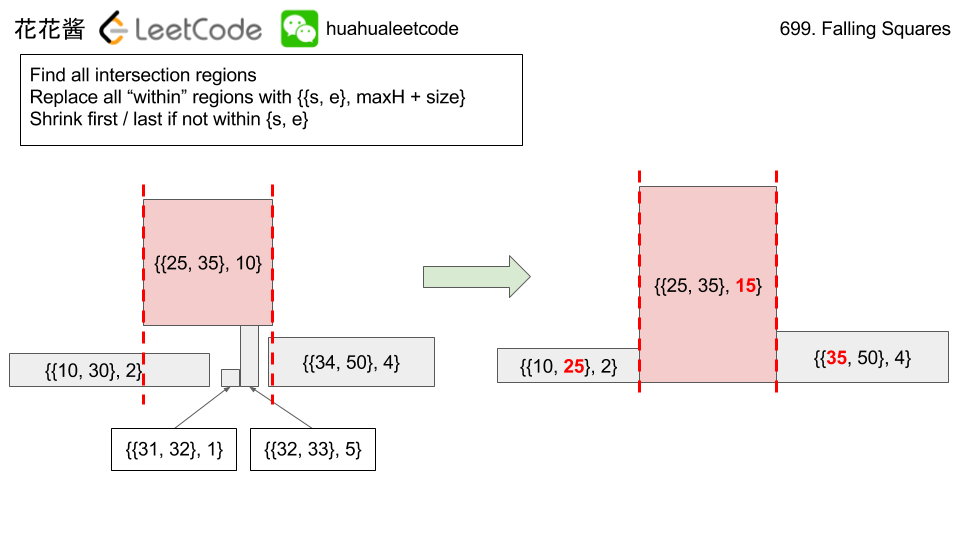# Posts tagged as “max”

Given a positive integer num consisting only of digits 6 and 9.

Return the maximum number you can get by changing at most one digit (6 becomes 9, and 9 becomes 6).

Example 1:

Input: num = 9669
Output: 9969
Explanation:
Changing the first digit results in 6669.
Changing the second digit results in 9969.
Changing the third digit results in 9699.
Changing the fourth digit results in 9666.
The maximum number is 9969.


Example 2:

Input: num = 9996
Output: 9999
Explanation: Changing the last digit 6 to 9 results in the maximum number.

Example 3:

Input: num = 9999
Output: 9999
Explanation: It is better not to apply any change.

Constraints:

• 1 <= num <= 10^4
• num‘s digits are 6 or 9.

## Solution: Greedy

Replace the highest 6 to 9, if no 6, return the original number.

Time complexity: O(1)
Space complexity: O(1)

# Problem

Given an integer array, find three numbers whose product is maximum and output the maximum product.

Example 1:

Input: [1,2,3]
Output: 6


Example 2:

Input: [1,2,3,4]
Output: 24


Note:

1. The length of the given array will be in range [3,104] and all elements are in the range [-1000, 1000].
2. Multiplication of any three numbers in the input won’t exceed the range of 32-bit signed integer.

# Idea:

Find the top 3 numbers t1, t2, t3, and bottom 2 numbers, b1, b2.

If all numbers are positives,  answer must be t1 * t2 * t3.

Since the number can go negative, the answer must be either t1*t2*t3 or b1 * b2 * t1, if b1 and b2 are both negatives.

ex. nums: [5, 1, -6, 3, -1]

t1, t2, t3: 5, 3, 1

b1, b2: -6, -1

t1 * t2 * t3 = 15

t1 * b1 * b2 = 30

# Solution 1: Manual Tracking

Time complexity: O(n)

Space complexity: O(1)

# Solution 2: Sorting

Time complexity: O(nlogn)

Space complexity: O(1)

# Solution 3: Two Heaps (Priority Queues)

Time complexity: O(nlog3)

Space complexity: O(2 + 3)

# Problem

https://leetcode.com/problems/third-maximum-number/description/

Given a non-empty array of integers, return the third maximum number in this array. If it does not exist, return the maximum number. The time complexity must be in O(n).

Example 1:

Input: [3, 2, 1]

Output: 1

Explanation: The third maximum is 1.


Example 2:

Input: [1, 2]

Output: 2

Explanation: The third maximum does not exist, so the maximum (2) is returned instead.


Example 3:

Input: [2, 2, 3, 1]

Output: 1

Explanation: Note that the third maximum here means the third maximum distinct number.
Both numbers with value 2 are both considered as second maximum.

# Solution: Set

Time complexity: O(n)

Space complexity: O(1)

C++

Python3

Problem:

Find the contiguous subarray within an array (containing at least one number) which has the largest sum.

For example, given the array [-2,1,-3,4,-1,2,1,-5,4],
the contiguous subarray [4,-1,2,1] has the largest sum = 6.

Idea:

DP

Solution:

C++

Problem:

On an infinite number line (x-axis), we drop given squares in the order they are given.

The i-th square dropped (positions[i] = (left, side_length)) is a square with the left-most point being positions[i] and sidelength positions[i].

The square is dropped with the bottom edge parallel to the number line, and from a higher height than all currently landed squares. We wait for each square to stick before dropping the next.

The squares are infinitely sticky on their bottom edge, and will remain fixed to any positive length surface they touch (either the number line or another square). Squares dropped adjacent to each other will not stick together prematurely.

Return a list ans of heights. Each height ans[i] represents the current highest height of any square we have dropped, after dropping squares represented by positions, positions, ..., positions[i].

Example 1:

Example 2:

Note:

• 1 <= positions.length <= 1000.
• 1 <= positions[i] <= 10^8.
• 1 <= positions[i] <= 10^6.

Idea:

Range query with mapSolution:

C++ map

C++ vector without merge

C++ / vector with merge (slower)

Related Problems:

Mission News Theme by Compete Themes.